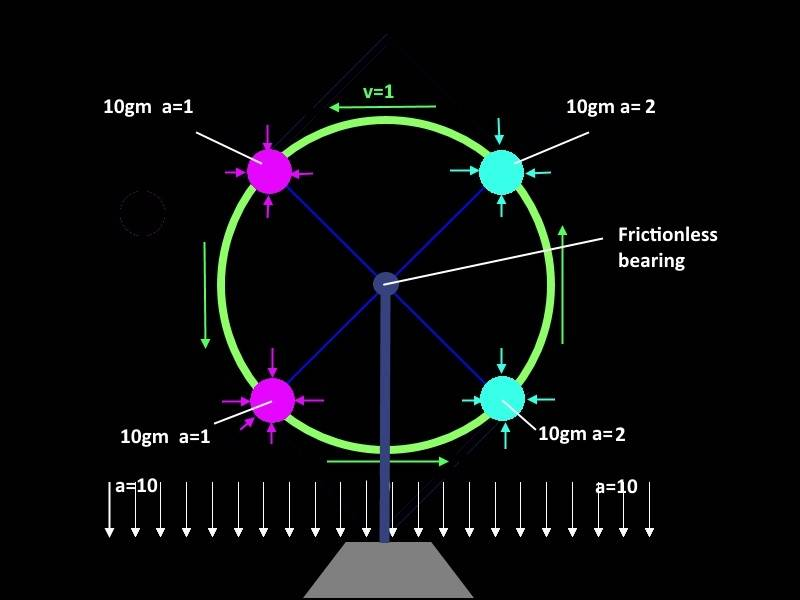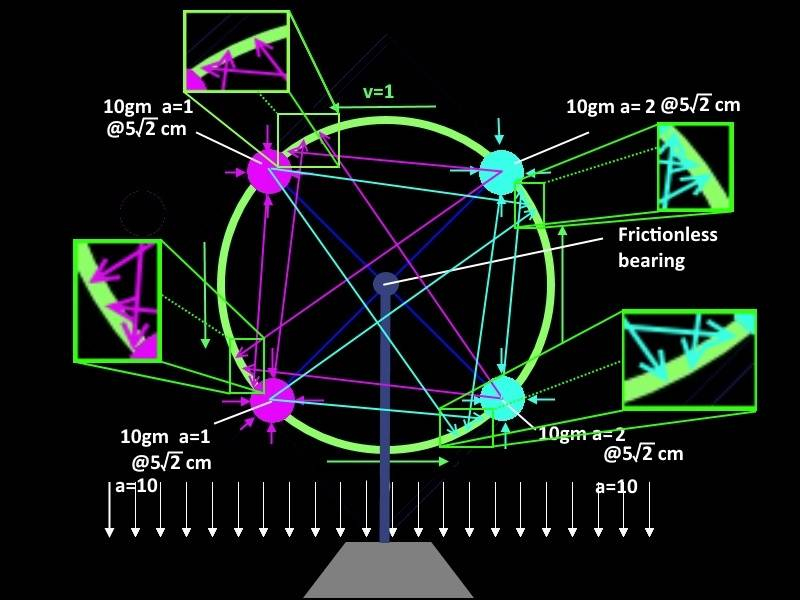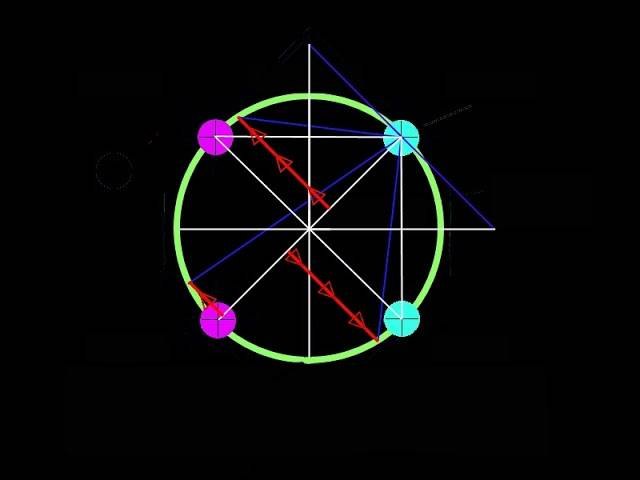# Change in angular velocity of the following system

The pink spheres cause an acceleration of 1 cm per second squared towards themselves.

The aqua spheres cause an acceleration of 2 cm per second towards themselves.

All spheres have the same mass.

The spheres are attached to a massless, yet mysteriously solid perfectly circular green ring, equidistant from the closest spheres (pi/2 from one another).

The 10 cm diameter green ring is rotating at a velocity of 1 cm per second.

Everything except the pedestal experiences an acceleration of 10 cm per second squared downwards (in the direction from the bearing towards the pedestal). The pedestal is fixed in place (does not accelerate downwards).

The ring is held up from the pedestal by a post with a frictionless bearing.At what rate does the velocity of the ring change over time if acceleration transmission is instantaneous?

At what rate does the velocity of the ring change over time if acceleration transmission is relativistic?

Last edited:

mfb
Mentor
What is this, a strange homework problem? An attempt to violate conservation of energy?
It is certainly not mathematics, but I'm unsure where to put the thread.
The pink spheres cause an acceleration of 1 cm per second squared towards themselves.

The aqua spheres cause an acceleration of 2 cm per second towards themselves.

All spheres have the same mass.
That doesn't make sense. Acceleration depends on distance, and for the same mass the gravitational force is the same.

What is this, a strange homework problem? An attempt to violate conservation of energy?
It is certainly not mathematics, but I'm unsure where to put the thread.
It's a math question about an exaggerated fictional scenario. I wouldn't call it physics. It will help me understand a concept.
That doesn't make sense. Acceleration depends on distance.....
I thought of adding that later, it should say (although I'm still curious about the mathematical solution with non proportional acceleration!):
The pink spheres cause an acceleration of 1 cm per second squared towards themselves at a distance of 5√2cm.

The aqua spheres cause an acceleration of 2 cm per second towards themselves at a distance of 5√2cm.

The arrows point to "where" (greatly exaggerated) the acceleration is towards in the relativistic scenario.I'm assuming similar scenarios come up a lot, so thought there would be some ready made formulas to plug values into to determine the acceleration.

Last edited:
mfb
Mentor
I'm assuming similar scenarios come up a lot
Not really.

If you want to use the physical laws of our universe, the correct treatment is general relativity. Calculating that system in general relativity is way beyond your knowledge. There are also no perfectly rigid materials any more.
You can use Newton's laws as approximation, then you can ignore all internal forces and just calculate the net torque on the wheel.

If you want to use the physical laws of our universe, the correct treatment is general relativity. Calculating that system in general relativity is way beyond your knowledge.
Ehh, I want to calculate torque on the wheel using fictional forces to illustrate a point.

I was going to use the following approximation (more or less, pressed for time):

For the shortest acceleration distance:
1) Constrain 0< s < 5√2 cm / c
2) τ = v / 10π
3) calculate s by using 10 sin(π/4 - τs/2)- c s /100 = 0 (c in cm) // ??????????
4) Use s to determine the distance from accelerating body
5) calculate magnitude of acceleration vector
6) multiply by sin (π/4 +τs/2).... now you have a relativistic approximation
....

You can use Newton's laws as approximation, then you can ignore all internal forces and just calculate the net torque on the wheel.
You can't. Instantaneous transmission of forces results in no net torque on the wheel.

Last edited:
mfb
Mentor
Ehh, I want to calculate torque on the wheel using fictional forces to illustrate a point.
The point is pointless. It's like 5 random numbers you see in 5 different places. Sure you can add them, but how would that give any interesting result.
You can't. Instantaneous transmission of forces results in no net torque on the wheel.
As I said, you can ignore all internal forces.... net torque can still come from external forces, like gravity.

Dale
Mentor
2021 Award
At what rate does the velocity of the ring change over time if acceleration transmission is instantaneous?
0

•CWatters and Matt Benesi
0
That cracked me up. Thanks for the morning happiness.

The point is pointless.
I'm assuming you know how cool that comment is.
It's like 5 random numbers you see in 5 different places. Sure you can add them, but how would that give any interesting result.
If you input non-random numbers from a similar (actual) physical problem the result is interesting. The results set constraints on what certain experimental setups can detect within their margins of error. It shows that claims that the experiments detected these things are false.

I'll post the results later, and try to explain some of the reasoning behind bringing up this problem in the first place.

mfb
Mentor
Please keep our policy on personal speculation in mind.

Dale
Mentor
2021 Award
That cracked me up. Thanks for the morning happiness.
You are welcome!

If it isn't obvious to you why 0 is correct then I would be glad to explain.

You are welcome!

If it isn't obvious to you why 0 is correct then I would be glad to explain.
lol. Leave it open for someone who doesn't know the answer to figure out.

Please keep our policy on personal speculation in mind.
It's never personal when it's math. It's just right, wrong, or undefined.

Dale
Mentor
2021 Award
lol. Leave it open for someone who doesn't know the answer to figure out.
OK.

I dropped the downward acceleration for the following, just did a ring with 4 spherical masses. You can run this in WxMaxima if you want to play with it.

function usage in WxMaxima is:

fiterall(r,v,mass,accmult,iter,skippy)$with: r= radius of ring body v= tangential velocity of ring mass= "mass" of sphere to calculate acceleration from G (used G because I wanted to check something) accmult= multiplier for acceleration for aqua colored spheres iter= # of iterations to perform the homing function** set it > 100 for decent accuracy skippy= number of iterations between displaying iteration number (you can set this really high if you don't care about tracking calculation times) **each iteration of the homing function reduces error in position of acceleration source Input the following code (between lines of "**************") into WxMaxima for function declaration: ********************************************************************************************************* fpprec:128$fpprintprec:32$fiterall(r,v,mass,accmult,iter,skippy):= [pi:bfloat(%pi),acc1:mass*6.67408b-14*(100b0/r)^2b0, acc2:acc1*accmult, c:299792458b0*100b0, t:v/(2b0*r*pi*2b0), i:1,ss:r*sqrt(2b0)/c,zz: r*2b0*sin(pi/4b0-ss*t)-c*s, steppy:ss*.1b0, for i thru iter do[zold:abs(zz), if (zz>0b0) then [ss:ss+steppy] else [ss:ss-steppy], zz: r*2b0*sin(pi/4b0-ss*t)-c*ss, if (abs(zz)>zold) then steppy:steppy/2b0, if integerp(i/skippy) then disp(sconcat(" iterations: ",(i)))], disp(sconcat(" Short Error (from perfect time): ", float(zz))), smalltime:ss, i:1,ss:r*2b0/c,zz: r*2b0*sin(pi/2b0-ss*t)-c*s, steppy:ss*.1b0, for i thru iter do[zold:abs(zz), if (zz>0b0) then [ss:ss+steppy] else [ss:ss-steppy], zz: r*2b0*sin(pi/2b0-ss*t)-c*ss, if (abs(zz)>zold) then steppy:steppy/2b0, if integerp(i/skippy) then disp(sconcat(" iterations: ",(i)))], disp(sconcat(" Diag. Error (from perfect time): ", float(zz))), diagtime:ss, i:1,ss:r*sqrt(2b0)/c,zz: r*2b0*sin(pi/4b0+ss*t)-c*s, steppy:ss*.1b0, for i thru iter do[zold:abs(zz), if (zz>0b0) then [ss:ss+steppy] else [ss:ss-steppy], zz: r*2b0*sin(pi/4b0+ss*t)-c*ss, if (abs(zz)>zold) then steppy:steppy/2b0, if integerp(i/skippy) then disp(sconcat(" iterations: ",(i)))], disp(sconcat(" Long Error (from perfect time): ", float(zz))), longtime:ss, shortacc:(r^2b0*2b0/((smalltime*c)^2b0))*sin(t*2*smalltime+pi/2), diagacc:(r^2b0*4b0/((diagtime*c)^2b0))*sin(-t*2*diagtime+pi), longacc:(r^2b0*2b0/((longtime*c)^2b0))*sin(-t*2*longtime/c+pi/2), aqua1:(shortacc+diagacc)*acc1-longacc*acc2, aqua2:shortacc*acc2+(diagacc-longacc)*acc1, pink3:acc2*(shortacc+diagacc)-longacc*acc1, pink4:shortacc*acc1+acc2*(diagacc-longacc), total:aqua1+aqua2+pink3+pink4, disp(" "), disp(sconcat(" acceleration: ", float(acc1))), disp(sconcat(" total a: ", float(total))), disp(sconcat(" smalltime: ", float(smalltime))), disp(sconcat(" diagtime: ", float(diagtime))), disp(sconcat(" longtime: ", float(longtime))) ]$
*********************************************************************************************************

Then run it with the following command to get some results:

fiterall(5b0,1b0,1b0,1.000b0,580,190)$At v~.3289948*c, tangential acceleration of the ring is equal to acceleration towards the test masses (when all test masses have the same acceleration towards themselves). Dale Mentor 2021 Award In the Newtonian case the angular acceleration is 0. I guess you are treating the relativistic case. If so then it can only decelerate, not accelerate. The ring is rotating counterclockwise. Assume equal masses, equal acceleration. The point the masses are attracted to is shifted like (greatly exaggerated):We only add the tangential components to the acceleration of the ring. Dale Mentor 2021 Award It cannot happen that way. Any relativistic field will radiate away the angular momentum, not gain more out of nowhere. I wanted to see what relativistic propagation of a gravity like acceleration would do in that scenario. Basically, if the gravity like acceleration propagates at c, and there are no other forces acting on the ring, the ring will experience an angular acceleration if all 4 sources are the same "mass". Maybe frame dragging, or some other effect cancels out the acceleration in real life. Maybe not. I corrected the WxMaxima code: fpprec:128$fpprintprec:32$fiterall(r,v,mass,accmult,iter,skippy):=[ pi:bfloat(%pi), c:299792458b0*100b0, t:v/(2b0*r*pi*2b0), i:1,ss:r*sqrt(2b0)/c,zz: r*2b0*sin(pi/4b0-ss*t)-c*s, steppy:ss*.1b0, for i thru iter do[zold:abs(zz), if (zz>0b0) then [ss:ss+steppy] else [ss:ss-steppy], zz: r*2b0*sin(pi/4b0-ss*t)-c*ss, if (abs(zz)>zold) then steppy:steppy/2b0, if integerp(i/skippy) then disp(sconcat(" iterations: ",(i)))], disp(sconcat(" Short Error (from perfect time): ", float(zz))), smalltime:ss, i:1,ss:r*2b0/c,zz: r*2b0*sin(pi/2b0-ss*t)-c*s, steppy:ss*.1b0, for i thru iter do[zold:abs(zz), if (zz>0b0) then [ss:ss+steppy] else [ss:ss-steppy], zz: r*2b0*sin(pi/2b0-ss*t)-c*ss, if (abs(zz)>zold) then steppy:steppy/2b0, if integerp(i/skippy) then disp(sconcat(" iterations: ",(i)))], disp(sconcat(" Diag. Error (from perfect time): ", float(zz))), diagtime:ss, i:1,ss:r*sqrt(2b0)/c,zz: r*2b0*sin(pi/4b0+ss*t)-c*s, steppy:ss*.1b0, for i thru iter do[zold:abs(zz), if (zz>0b0) then [ss:ss+steppy] else [ss:ss-steppy], zz: r*2b0*sin(pi/4b0+ss*t)-c*ss, if (abs(zz)>zold) then steppy:steppy/2b0, if integerp(i/skippy) then disp(sconcat(" iterations: ",(i)))], disp(sconcat(" Long Error (from perfect time): ", float(zz))), longtime:ss, squareacc:sqrt(r*sqrt(2b0)/(r*sqrt(2b0)-2b0*mass*6.67408b-14/c^2b0)) *mass*6.67408b-14*(100b0/(r*sqrt(2b0)))^2b0, diagonalacc:sqrt(r*2b0/(r*2b0-2b0*mass*6.67408b-14/c^2b0)) *mass*6.67408b-14*(100b0/(r*2b0))^2b0, sr:smalltime*c, shortacc:(sqrt(sr/(sr-2b0*mass*6.67408b-14/c^2b0)) *mass*6.67408b-14*(100b0/sr)^2b0) *sin(t*2*smalltime+pi/2), sr:diagtime*c, diagacc:(sqrt(sr/(sr-2b0*mass*6.67408b-14/c^2b0)) *mass*6.67408b-14*(100b0/sr)^2b0) *sin(-t*2*diagtime+pi), sr:longtime*c, longacc:(sqrt(sr/(sr-2b0*mass*6.67408b-14/c^2b0)) *mass*6.67408b-14*(100b0/sr)^2b0) *sin(-t*2*longtime/c+pi/2), aqua1:(shortacc+diagacc)-longacc*accmult, aqua2:shortacc*accmult+(diagacc-longacc), pink3:accmult*(shortacc+diagacc)-longacc, pink4:shortacc+accmult*(diagacc-longacc), total:aqua1+aqua2+pink3+pink4, disp(" "), disp(sconcat(" square acc: ", float(squareacc))), disp(sconcat(" diagonal acc: ", float(diagonalacc))), disp(sconcat(" total acc: ", float(total))), disp(sconcat(" smalltime: ", float(smalltime))), disp(sconcat(" diagtime: ", float(diagtime))), disp(sconcat(" longtime: ", float(longtime))) ]$

squareacc and diagonalacc are the Schwarzschild approximation accelerations for the ring at rest... which is sort of pointless, because they cancel out at rest.

So, assume that the ring is super small, Planck scale small, and its momentum ramps up really quickly at that level. It would be like a tiny little gyroscope. Neat huh?

Dale
Mentor
2021 Award
. Basically, if the gravity like acceleration propagates at c
It isn't that simple. That is why general relativity was developed. Einstein tried to do exactly what you are describing, but found that it leads to an inconsistent theory.

In the simplest consistent relativistic theory of gravity, GR, the angular momentum of the system radiates away in the gravitational waves.

•mfb
mfb
Mentor
If the four masses are equal, I'm not sure if that system has a variable quadrupole moment. It could rotate without radiating, in the same way a ring will do.

•Matt Benesi and Dale
Dale
Mentor
2021 Award
If the four masses are equal, I'm not sure if that system has a variable quadrupole moment. It could rotate without radiating, in the same way a ring will do.
Good point. As you say, it isn't obvious to me that there is any GW emission, but it is obvious that any change in angular momentum must be negative.

Why?

Imagine observers in other locations.

mfb
Mentor
It does not matter where the observers are. All observers will agree on whether gravitational waves are emitted, and no observer will see a speedup of angular velocity.

Dale
Mentor
2021 Award
Nor does it matter how fast they are travelling (assuming an asymptotically flat background). They may disagree on the magnitude of the change in angular velocity, but not the sign.

•Matt Benesi
Would frame dragging be a stretch to explain this at these distances/velocities/masses?

mfb
Mentor
What do you mean by "this"?

No.

lol

Dale
Mentor
2021 Award
These calculations are beyond me right now, and I don't have a well informed intuition about which effects will be larger. Both gravitational waves and frame dragging may be present.

These calculations are beyond me right now, and I don't have a well informed intuition about which effects will be larger. Both gravitational waves and frame dragging may be present.
Do gravitational waves emit from symmetric bodies rotating around an axis of symmetry?